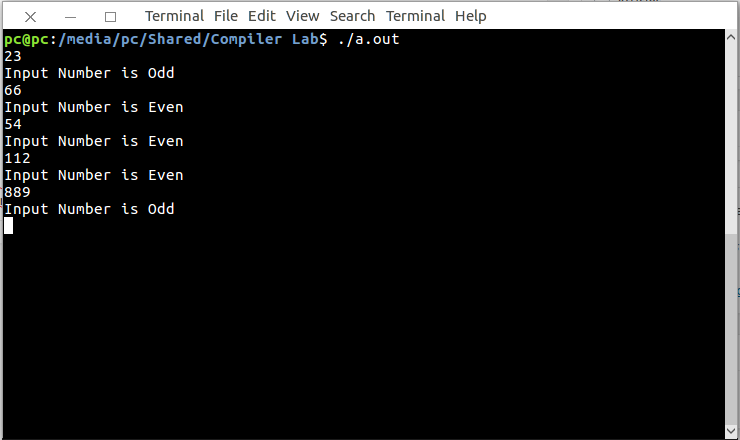Open In App

# Lex program to check whether input number is odd or even

Lex is a computer program that generates lexical analyzers. Lex reads an input stream specifying the lexical analyzer and outputs source code implementing the lexer in the C programming language.

The commands for executing the lex program are:

```lex abc.l (abc is the file name)
gcc lex.yy.c -ll
./a.out
```

Let’s see lex program to check whether input number is odd or even.

Examples:

```Input :
22
Output :
Input Number is Even

Input :
53
Output :
Input Number is odd
```

Below is the implementation:

 `/% Lex Program to check whether ``      ``- input number is odd or even. %/`` ` `% {``   ``int` `i;``% }`` ` `%%``[0-9]+ {i = ``atoi``(yytext); `` ` `if``(i%2==0) `` ``printf``(``"Input Number is Even"``); `` ` `else` ` ``printf``(``"Input Number is Odd"``);``};`` ` `%%`` ``int` `main()``  ``{``    ``yylex();``    ``return` `1;``  ``}`

Output: